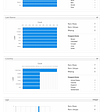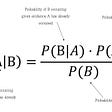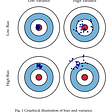# Understanding Different Types of Distributions You Will Encounter As A Data Scientist

As a Data Scientist, you will be looking at a lot of data. While that may be common sense, you also need to understand that not all data is the same. Some of your data sets may be continuous distributions while others may be discrete. Some columns of your table may follow a Gaussian distribution while others may be exponential. Due to numerous types of data distributions possible, it is important to establish a solid understanding of the most common types and be familiar of the situations where you are likely to see one.

# Discrete vs Continuous Distributions

A simple way to understand if your data is discrete or continuous is the answer the following question: Are the number outcomes finite?

If the answer to the above is yes, then you have a discrete dataset. Otherwise, you likely have a continuous dataset.

But what does finite mean, exactly? Well, if you imagine a roll of a fair dice, you know that you have exactly 1/6 chance of rolling a 1, 2, 3, 4, 5, or 6. These values are finite because there is no chance of getting a 1.2 or 2.6 or other smaller value that isn’t exactly 1 or 2. These outcomes are a set of values rather than a continuous length of values.

An example of a continuous distribution would be weather. On any given day, the weather channel may say it is 62 degrees Fahrenheit. The actual temperature may not be exactly 62. It could be 62.01, 62.001, or even 62.00000001. All these values are different from the other and as such as referred to as a continuous range of values.

# Examples of Discrete Distributions

A few common examples of discrete distributions include the Bernoulli Distribution, the Poisson Distribution, and the Uniform Distribution.

The Bernoulli Distribution essentially represents the probability of success of an experiment. An example would be tossing a coin where the 2 outcomes are given a probability of occurrence.

The Poisson Distribution represents the probability of n events in a given time period when the overall rate of occurrence is constant. In simpler terms, it gives you a probability of how often something might happen. We can use the amount of mail you receive everyday. A Poisson Distribution would give you the probability of how many pieces of mail you receive each day.

The Uniform Distribution is the simplest distribution to explain. Is is when all the outcomes are equally likely.

# Example of Continuous Distribution

The Gaussian or normal distribution is the most common distribution that you will come across. This follows a bell shape, which is the name you may recognize it by, and is found in many real world data, such as height and weight.

# Conclusion

There are many different types of distributions and while each one describes a different situation, the normal distribution serves as the foundation within the Data Science community. Since this is the most common distribution that is naturally occurring, it is vital to begin your understanding of distributions with the Gaussian distribution. To begin your journey, please feel free to check out the links below that will help you learn.

https://www.analyticsvidhya.com/blog/2017/09/6-probability-distributions-data-science/

--

--

--

## More from MyTake

Smart Insight Communities

## A Beginner’s Guide to Performing RFM Analysis with Python## The OSINT Toolkit!## Data Science vs Data Engineering## Is SFrame better than Pandas?:## We’re tracking the most up-to-date information about the coronavirus in North Carolina.## The Budget Guru || my publications## Another Wave, the Same Mistakes## Practical Applications of Machine Learning to Supply Chain Planning: A Few Suggestions## Akshay Sharma

Data scientist with consulting and public accounting experience, and a CPA background at one of the largest accounting firms.

## Updating Our Beliefs in Light Of New Information — Bayes' Theorem## Google’s Data Analytics Certificate worth it?## Going from Zero to Data Hero: Navigating Data Roles Throughout Your Career## Beyond Bias & Variance